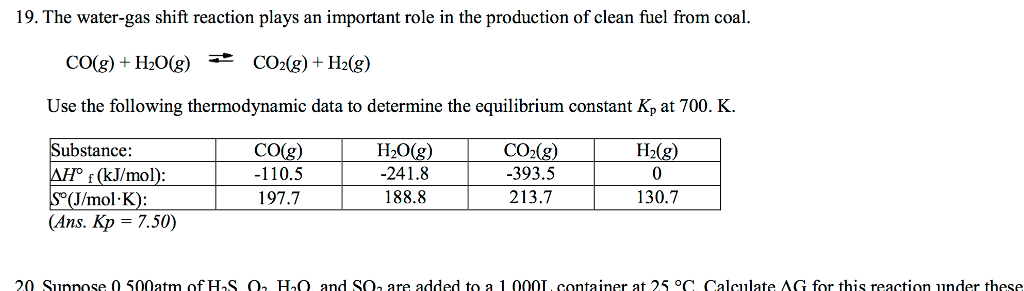# Question & Answer: The water-gas shift reaction plays an important role in the production of clean fuel from coal……The water-gas shift reaction plays an important role in the production of clean fuel from coal. CO(g) + H_2O(g) leftrightarrows CO_2(g) + H_2(g) Use the following thermodynamic data to determine the equilibrium constant K_p at 700. K.

Enthalpy of reaction = sum of enthalpy of products- sum of enthalpy of reactants

Don't use plagiarized sources. Get Your Custom Essay on
Question & Answer: The water-gas shift reaction plays an important role in the production of clean fuel from coal……
GET AN ESSAY WRITTEN FOR YOU FROM AS LOW AS \$13/PAGE

For the reaction, deltaH= 1* deltaH of CO2+1* deltaH of H2- { 1* deltaH of CO+1*deltaH of H2O}

1,1,1 and 1 are coefficients of CO2, H2, CO and H2O respectively.

deltaH= 1*-393.5+1*0 –{ 1*-110.5+1*(-241.8)}=-41.2 KJ/mole

similarly, deltas= 213.7*1+1*130.7- ( 1*197.7+1*188.8)=-42.1 J.mol.K

deltaG= deltaH-T*delts = -41.2*1000+700*42.1 =-11730 J/mole

but deltaG=-RTlnK, K= equilibrium constant

-11730= -8.314*700*lnK

K= 7.5